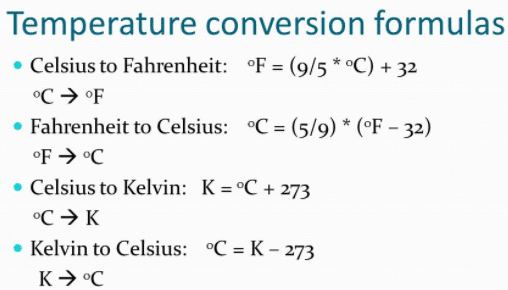Thermodynamics

# Temperature Conversion formulas and examplesIn this post, you’ll learn about Different temperature conversion Formulas.
This post includes:

• Celsius to Fahrenheit conversion formula
• Fahrenheit to Celsius conversion formula
• Celsius to the kelvin conversion formula
• Kelvin to Fahrenheit conversion formula
• Lots more

Let’s Dive right in…

### What are different scales of temperature?

There are three different Temperature conversion scales, which are given in the list:

• Celsius scale
• Fahrenheit scale
• Kelvin scale

### 1.Temperature Conversion from Celsius to Kelvin scale:

The temperature T on the Kelvin scale can be obtained by adding 273 in the temperature C on the Celsius scale. Thus the formula to convert Celsius to kelvin is given as:

T(k) = 273 + C

Example: What will be the temperature on the Kelvin scale of temperature when it is 20 °C on the Celsius scale?

Solution:                                                                                                       C=20ºC

T=273+C

T=273+20

T=293 K

### 2.Temperature Conversion from Kelvin to Celsius scale:

The temperature on the Celsius scale can be found by subtracting 273 from the temperature on the kelvin scale. Thus formula to convert temperature from Kelvin to Celsius is given by:

C =T(K) – 273

Example: Change 300 k on the Kelvin scale into the Celsius scale of temperature.

Solution:

T=300 K

Since C=T(K) – 273

Therefore:  C=(300-273)°C

C=27°C

### Temperature conversion from Celsius to Fahrenheit scale

Since 100 divisions on the Celsius scale are equal to 180 divisions on the Fahrenheit scale. Therefore,each division on the Celsius scale is equal to 1.8 divisions on the Fahrenheit scale. Moreover,0°C corresponds to 32°F.
Therefore: F=9/5(C +32)
Here F is the temperature on the Fahrenheit scale and C on the Celsius scale.
Example: Convert 50°C  on the Celsius scale to the Fahrenheit temperature scale.
Solution:

C=50 °C

F=1.8×(C + 32)

F=1.8×(50 +32)

F=122°F

### Temperature conversion from Fahrenheit to Celsius scale:

we can convert temperature from Fahrenheit to Celsius scale with the given formula as:

C =F – 32/1.8

Example: Convert 100 °F into the temperature on the Celsius scale.

Solution:

F= 100°F

Since C=F – 32/1.8

=100-32/1.8

C= 37.8  °C